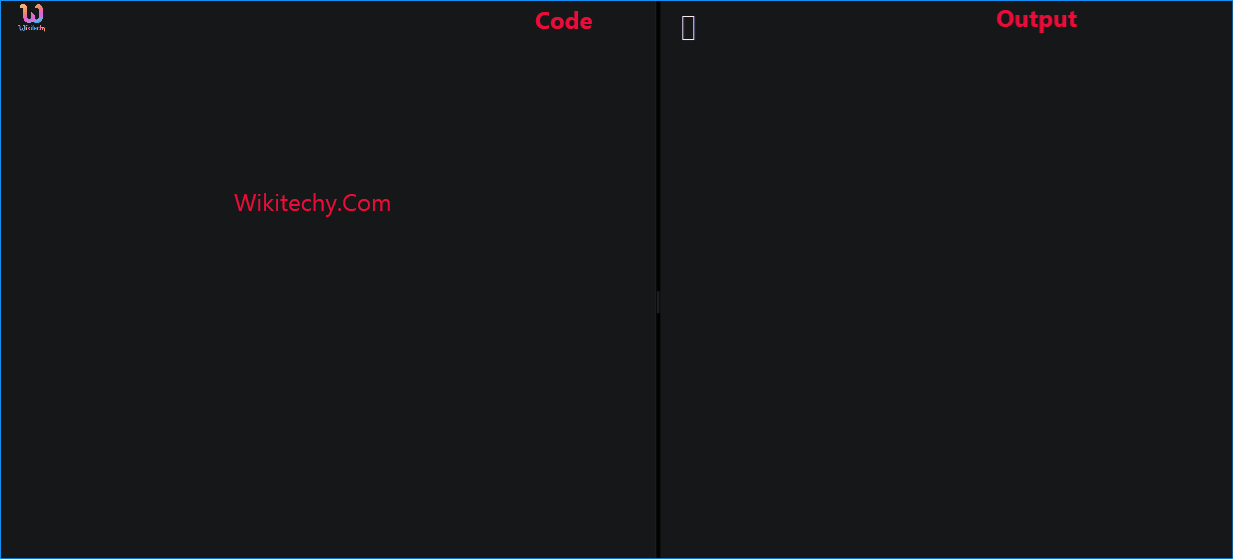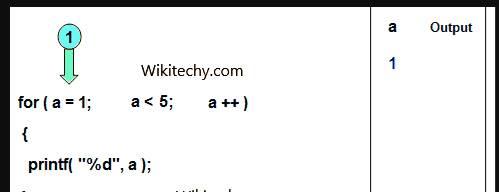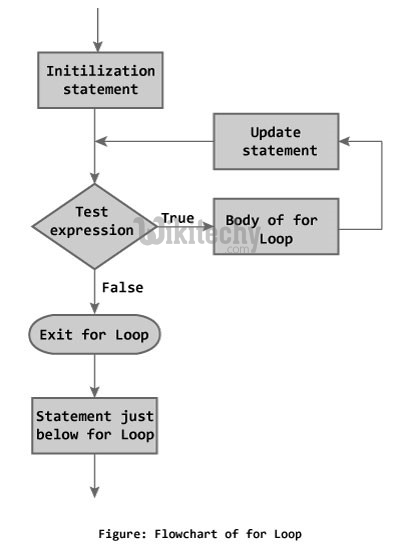# C++ For Loop - Learn C++ , C++ Tutorial , C++ programmingLearn c++ - c++ tutorial - c++ for loop - c++ examples - c++ programs

• Loops are used in programming to repeat a specific block of code. In this, you will learn to create a for loop in C++ programming (with examples).learn c++ tutorials - for loop Example

• Loops are used in programming to repeat a specific block until some end condition is met.
• There are three type of loops in C++ programming:
• for loop
• while loop
• do...while loop
• In this, you are going to learn for loop only

## C++ for Loop Syntax

• A for loop is a repetition control structure that allows you to efficiently write a loop that needs to execute a specific number of times.
• where, only testExpression is mandatory.

## How for loop works?

• The initialization statement is executed only once at the beginning.
• Then, the test expression is evaluated.
• If the test expression is false, for loop is terminated. But if the test expression is true, codes inside body of for loop is executed and update expression is updated.
• Again, the test expression is evaluated and this process repeats until the test expression is false.

## Flowchart of for Loop in C++## Output

• In the program, user is asked to enter a positive integer which is stored in variable n(suppose user entered 5). Here is the working of for loop:
1. Initially, i is equal to 1, test expression is true, factorial becomes 1.
2. i is updated to 2, test expression is true, factorial becomes 2.
3. i is updated to 3, test expression is true, factorial becomes 6.
4. i is updated to 4, test expression is true, factorial becomes 24.
5. i is updated to 5, test expression is true, factorial becomes 120.
6. i is updated to 6, test expression is false, for loop is terminated.
• In the above program, variable i is not used outside of the for loop. In such cases, it is better to declare the variable in for loop (at initialization statement).